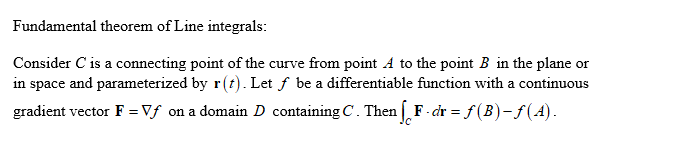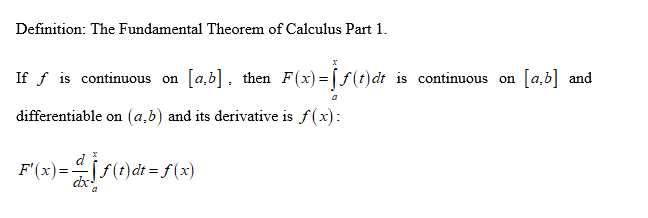# What is the Fundamental Theorem of line integrals? Explain how it relates to the Fundamental Theorem of Calculus.

Question
1 views

What is the Fundamental Theorem of line integrals? Explain how it relates to the Fundamental Theorem of Calculus.

check_circle

Step 1Step 2...

### Want to see the full answer?

See Solution

#### Want to see this answer and more?

Solutions are written by subject experts who are available 24/7. Questions are typically answered within 1 hour.*

See Solution
*Response times may vary by subject and question.
Tagged in